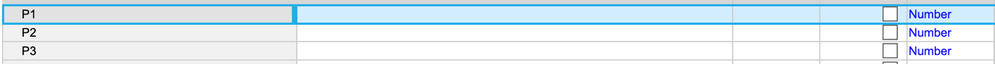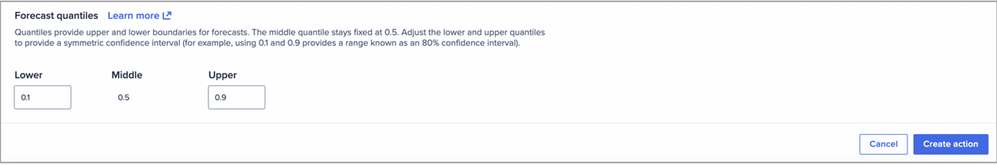# PlanIQ - Probabilistic forecasting using forecast quantilesedited December 2022

## Probabilistic forecasts & forecast quantiles

PlanIQ produces probabilistic forecasts. This means that PlanIQ algorithms output a distribution of possible values, rather than a single point forecast typically outputted by other solutions. This distribution can be divided into quantiles.

Applied to forecasting, quantiles help to address uncertainty in forecasted values. Quantiles define a prediction interval within which the actual value is likely to fall with a given probability. For example, a P10 quantile (P denotes Probability) indicates that the true observed value is expected to be lower than the forecasted value 10% of the time (i.e. probability of 10%), while a P90 quantile indicates that the true value is expected to be lower than the forecasted value 90% of the time. The difference between the P10 and P90 in this example defines an interval of 80%, which means that the probability of the true value falling between the forecasted values associated with the P10 and P90 quantiles is 80%. Increasing the difference between the quantiles would increase the interval and the probability.

Note that in the case of the median, or P50, 50% of the distribution falls on either side of the cut point. For quartiles, 25% of the distribution is in each interval at P25, P50, P75.

Selection of quantiles should be informed by business considerations about the relative costs associated with over and under forecasting.

## Selecting quantiles

The lower quantile (P10 in the example) can be used in instances where the cost of over-forecasting outweighs the cost of under-forecasting, such as when there are high costs associated with overproduction or overstocking. Use cases could include a manufacturing setting where there is a high cost of capital, as well as a contact center with labor-cost saving objective.

The upper quantile (P90 in the example) can be used in cases where the cost of under-forecasting outweighs the cost of over-forecasting. For example, in a retail setting with sufficient inventory space, the lost sales due to being understocked outweigh the cost of being overstocked, so forecasting at a higher quantile may be useful.

A P50 quantile, indicating that the true value is expected to be lower than the forecasted value 50% of the time, provides a balance between concerns of over-forecasting and under-forecasting.

In cases where the historical data is highly volatile or doesn’t seem to follow any pattern, it could be challenging to fit a forecast model that produces accurate predictions. Users may find the P50 quantile forecast to consistently under- or over-predict throughout the forecast horizon. In such instances, using the lower or upper quantile forecast as the point forecast of choice could produce more accurate predictions. However, if you choose to use a quantile forecast other than P50, it is recommended to reassess this selection in subsequent forecast refresh cycles.

## Using Quantiles with PlanIQ

PlanIQ supports 3 forecast quantiles generated with a single forecast action. The middle quantile stays fixed at P50. The lower and upper quantiles can be configured by the user. By default, PlanIQ sets the lower and upper quantiles to P10 and P90.

There are two steps to include quantiles in PlanIQ output:

1. The forecast results module and associated import action must include line items “P1”, “P2”, and “P3”.1. The quantiles must be specified in the Forecast Action step.Got feedback on this content? Let us know in the comments below.

Contributing authors: Nitzan Paz, Christophe Keomanivong, Frankie Wolf, Timothy Brennan, Andrew Martin, and Evgenya Kontorovich.

Tagged:

•Hi Team - Is there a way that we can import advanced metrics such as MAPE, RMSE, MAE etc. into Anaplan along with the forecast action? This would help business users to make the right decisions.

•@NitzanP Can you help with the above question?

Thank you!

Ginger, Community Manager

•Hi @hareesharry. Thank you for your question. The metrics aren't included in the forecast action as we would like to avoid confusion when it comes to forecast results analysis. More specifically, there are nuances related to the way in which the metrics are defined and their applicability to certain scenarios at the item-level (see examples below), so we rather allow users to determine the analysis approach they want to take.

Examples:
1. MAPE is undefined if the observed (actual) value is zero, and would produce extreme values for near-zero observed values. Users can determine how they would like to treat such results.
2. Over how many periods should the metric be calculated.

On a related topic, note that users can export the backtest data and calculate metrics at the item-level before running a forecast action.

•@NitzanP Thank you for the response above. But I would make a world difference to client if we have accuracy specific details that would provide confidence in the forecast.